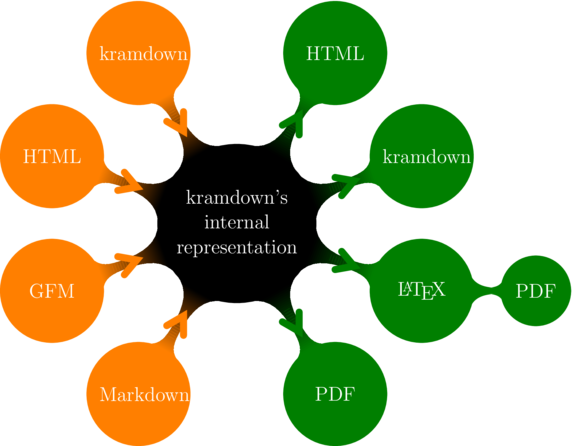# trying out kramdown

## kramdown seems awesome!

I heard and used kramdown already a long time ago. But I didn’t know they had such a good documentation on their website.

It is only after seeing this image that I went crazy.So I’ll try some math stuff here. I hope you don’t mind. (I don’t really know math)

## today I learned

everyone should know

$(a+b)^2 = a^2 + 2ab + b^2$

But in math there is always the hard way. Defined generally it looks different.

\begin{align*} \binom{n}{k}&=\frac{n!}{k!(n-k)!}\\ (a+b)^n &= \sum_{k=0}^n \binom{n}{k}\cdot a^{n-k}\cdot b^{k}\\ \end{align*}

And here starts the hilariousness

\begin{align*} (a+b)^2 = \binom{2}{0} \cdot a^{2-0} \cdot b^{0} + \binom{2}{1} & \cdot a^{2-1} \cdot b^{1} + \binom{2}{2} \cdot a^{2-2} \cdot b^{2} =\\ \frac{2!}{0!(2-0)!} \cdot a^2 \cdot b^0 + \frac{2!}{1!(2-1)!} & \cdot a^1 \cdot b^1 + \frac{2!}{2!(2-2)!} \cdot a^0 \cdot b^2 =\\ 1 \cdot a^2 \cdot 1 + 2 \cdot a \cdot b + 1 \cdot 1 & \cdot b^2 = a^2 + 2ab + b^2 \end{align*}

## how does the syntax look like?

Quite simple. One has only to put the latex code between the two dollar signs.

will render to

\begin{align*} (a+b)^n &= \sum_{k=0}^n \binom{n}{k}\cdot a^{n-k}\cdot b^{k} \end{align*}

Inline math is very similar.

renders to

$2^{3}+8=16$

## JS needed to render

Don’t forget to include the MathJax.js. I just took it from their website.

## edit

Actually the official MathJax from mathjax.org works way better.